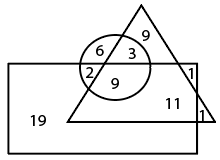Question 7

# In the given Venn diagram, the ‘circle’ represents ‘ladies’, the ‘triangle’ represents ‘teachers’, and the ‘rectangle’ represents ‘unmarried persons’. The numbers given in the diagram represent the number of persons in that particular category.How many married ladies are teachers?

Solution

Number of married ladies which are teachers = 3
$$\therefore$$ The correct answer is option C.

• Free SSC Study Material - 18000 Questions
• 230+ SSC previous papers with solutions PDF